# Solve Mountain Climber Rope Tension Problem for Exam Prep

• CelesteSeven

#### CelesteSeven

homework help! eek!

A mountain climber, in the process of crossing between two cliffs by a rope, pauses to rest. She weighs 665 N. As the drawing shows, she is closer to the left cliff than to the right cliff, with the result that the tensions in the left and right sides of the rope are not the same. Find the tensions in the rope to the left and to the right of the mountain climber.

IN THE PICTURE: there is a mountain climber suspended in the air almost halfway between 2 cliffs. It gives me two of the angles (the angle btwn the rope and the posts it is tied to on either end). One angle is 65 deg, the other angle on the opposite cliff is 80 deg.

So far, I drew 2 separate triangles. I made the y-axis of one of the triangles the 665N, and used trig to find the "length" or "tension" of the rope, but it keeps coming up to be incorrect. I know I am doing the trig right (I'm really great at trig and have taken through calc II so the math isn't the problem).

Any suggestions? This thing is due tonight and we have our midterm exam on Wednesday. Thanks!

This should be nothing more than simple resolving of forces. If you can post any working perhaps that would help in a diagnosis of where you have gone wrong.

A mountain climber, in the process of crossing between two cliffs by a rope, pauses to rest. She weighs 665 N. As the drawing shows, she is closer to the left cliff than to the right cliff, with the result that the tensions in the left and right sides of the rope are not the same. Find the tensions in the rope to the left and to the right of the mountain climber.

This is the original picture: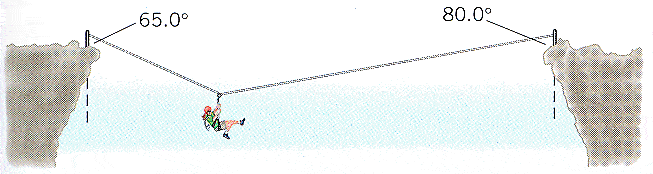And here is what I did (at least for the first part of the question; it wants 2 different answers -quite obviously- for the 2 different tensions).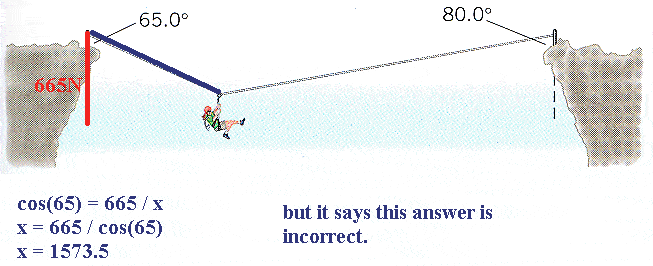The weight is the weight of the person, not the pole! The correct approach to this question is to consider the forces acting on the person. Resolve the tensions into horizontal and vertical components, then consider the resultant forces on the person in the horizontal and vertical directions.

we haven't done much with tenstion, Cristo, so I'm still as confused. all I can think of to do to draw the forces according to the person is to draw a straight line down from her (her force of weight) being 665N. But then I have a triangle with a another line drawn down (which seems obsolete).

so if I draw a line on the +y axis, that being the natural force, I get 665N that way. But that's the same triangle, just upside-down. am I getting warmer?

we haven't done much with tenstion, Cristo, so I'm still as confused. all I can think of to do to draw the forces according to the person is to draw a straight line down from her (her force of weight) being 665N.
Yup, that's right
But then I have a triangle with a another line drawn down (which seems obsolete).

so if I draw a line on the +y axis, that being the natural force, I get 665N that way.
This isn't correct. If this was on a surface, then yes, there would be a contact force but we are not.

Consider the point at which the person is hanging. There are three forces acting on her; her weight (which you corectly identified as being vertically downwards) and two tensions, each acting on the person at a different angle. The first step is to mark the angles near the person on the diagram.

Now, each of these tensions has a horizontal and vertical component. For each tension, you can draw a triangle consisting of the tension (as the hypotenuse) and the vertical and horizontal components (as the other two sides) You know one of the angles, and so can use trigonometry to find the other two sides.

See if you can get here, post your answers, and we'll go from there.

Now, each of these tensions has a horizontal and vertical component. For each tension, you can draw a triangle.QUOTE]

I don't quite understand that. But here's what I've done.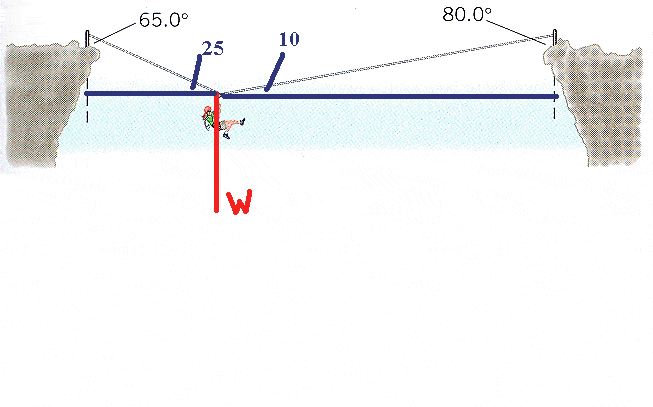and then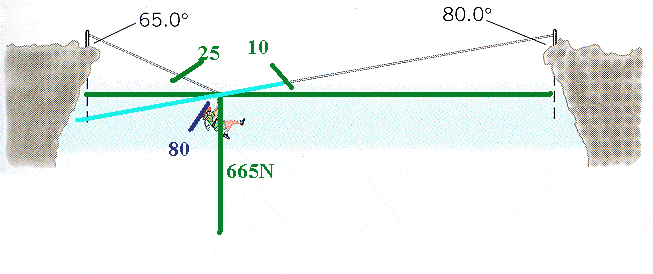anyone? time is running outdo you know what the correct answer is?

no, I don't. it's an online homework. I'd like to get the answer right before 11:30pm est (tonight) because that's when it's due. i can always go tomorrow (before the exam) and have the TA help me and explain it to me, but that doesn't do good for me point wise (and I really need them) and I also need to understand.

i don't want it spelled out to me (that's cheating) but I've tried many things and I still can't seem to get it and have only 2 attempts left.

k, well all you have to do is sum the forces in the x and y directions...Im guessing someone has already told you this and youre confused?

Have you learned how to do this...$$\sum \\F_x=0$$&$$\sum\\F_y=0$$? That is the sum of the forces in the x and y directions must equal zero?

Also, do you know how to resolve the forces of tension into their respective x and y components?

Or do you have to do it using trig?

i do know about the sum of forces. and I understand x and y components and how they are seperate.
i do not know how to resolve forces... or even what that means (and I go to class and pay attention, as well).
Our TA is a bit sloppy when it comes to giving us the correct problems though, unfortunately.

I draw triangles with one side being the weight, but I keep getting the same (wrong) answers.

I've tried this (enter in correct angle markings)... basically what I described. have any guidance for me?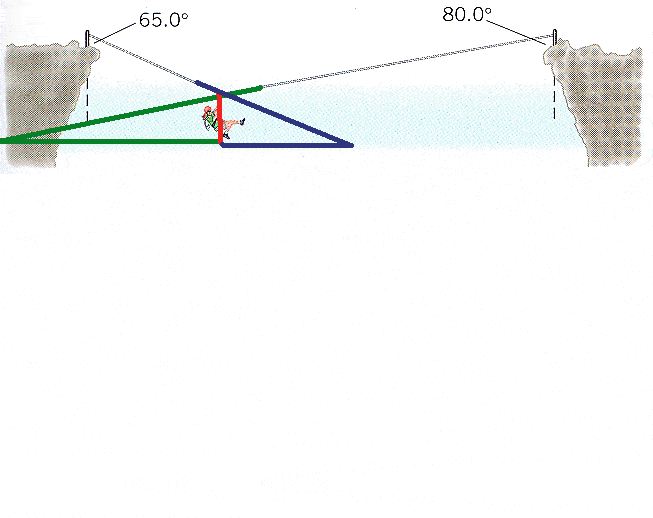no, I don't. it's an online homework. I'd like to get the answer right before 11:30pm est (tonight) because that's when it's due. i can always go tomorrow (before the exam) and have the TA help me and explain it to me, but that doesn't do good for me point wise (and I really need them) and I also need to understand.

i don't want it spelled out to me (that's cheating) but I've tried many things and I still can't seem to get it and have only 2 attempts left.
I think cristo hinted it out very good for you. Isolate the joint where the climber is hanging from. In the vertical direction her weight acts down, and the vertical componens of tension forces act up. Think Newton. In the horizontal direction, look at the horizontal components of the tensions, and what does Newton say about their sum??

No, I don't think we have to do it using trig.
But is there another way?
Just adding x and y components? I yield always the same incorrect answers. Not to mention the german midterm tomorrow. yikes, I am appreciating the help tonight!

Assuming "E" is the sigma...

EF = T - 665 = 0 (yes?)

The tension has both vertical and horizontal components, which add up to 665. But where are the components? I mean, the rope in the problem should be the hyp of the given triangle, right? That's what I keep assuming. But i yield the same answers.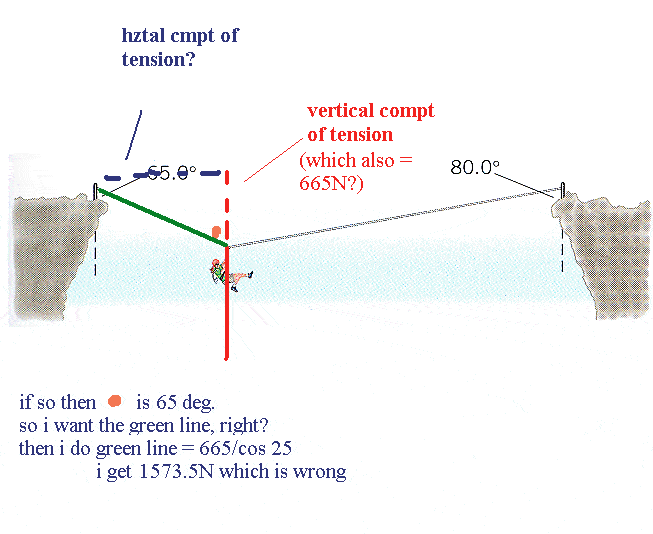BLAH! THAT COS25 SHOULD BE 65. I only typed that error on here, not in my calculator. In my calculator, i had 65.

There are Two Tensions, one for each rope. Each tension has a horizontal AND a vertical component.

Assuming "E" is the sigma...

EF = T - 665 = 0 (yes?)

The tension has both vertical and horizontal components, which add up to 665. But where are the components? I mean, the rope in the problem should be the hyp of the given triangle, right? That's what I keep assuming. But i yield the same answers.
In your summation equation in the vertical direction, there are 2 vert components of the tension forces, T1 and T2. Their vertical components sum to 665. Now look in the x direction for another equation using the hoizontal components of the 2 tension forces.

And yourn going to end up with two equations. Sum of the forces in the X direction, and sum of the forces in the Y direction, with two unknowns, you'll be able to solve.

thanks for all your help, but I'm afraid I still don't understand. it's frustrating enough, so I'm giving up on it.
thanks everyone for devoting your time. i appreciate it, and I think I understand the problem much better, even if I don't get it fully still.

thanks for all your help, but I'm afraid I still don't understand. it's frustrating enough, so I'm giving up on it.
thanks everyone for devoting your time. i appreciate it, and I think I understand the problem much better, even if I don't get it fully still.
Gelduld bringt Rosen!

hah, I believe you mean "Geduld"?

I know it does, however, this is due in an hour. I can always go tomorrow and have a TA spell it out for me (and I will do that). It's just a matter of 2 extra points tonight. I won't get them, but eh. Maybe I can make up for it on the midterm.